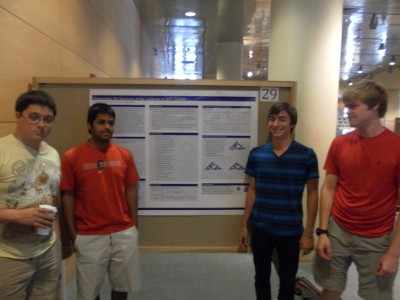# Determining the Spectrum of the Laplacian on 3N-Gaskets## Overview

The Laplacian is the central object of analysis on fractals. While most work on the Laplacian has been focused on computing the Laplacian spectrum on specific fractals, this group instead found the spectrum on an entire class of fractals—the 3N-Gaskets. This is the class of finitely ramified fractal 3N-Gons that are the attractors of iterated function systems containing 3N contraction mappings. For example, the 3-Gasket is the Sierpinski triangle.

The Laplacian on the fractal, and thus its eigenvalues, must be studied by examining the graph Laplacian on approximating graphs. The group found a function R(z) relating the Laplacian eigenvalues on consecutive graph approximations. They used R(z) along with the eigenvalues of the Laplacian on graph approximations to derive part of the spectrum on the next level. The rest of the spectrum was found using an “exceptional set”— a set of values which the group provided for arbitrary N. By repeating this infinitely many times, the spectrum of the Laplacian on the fractal could be found.

arXiv.org